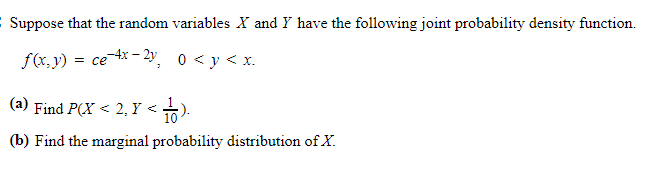# Question Suppose that the random variables X and Y have the following joint probability density function. f(x,y) = ce-4x – 2y, 0 <y<x. (a) Find P(X<2,Y<1 10 (b) Find the marginal probability distribution of X.LZWQ5H The Asker · Probability and StatisticsTranscribed Image Text: Suppose that the random variables X and Y have the following joint probability density function. f(x,y) = ce-4x – 2y, 0
More
Transcribed Image Text: Suppose that the random variables X and Y have the following joint probability density function. f(x,y) = ce-4x – 2y, 0